# Python 实现的随机森林

##### 什么是随机森林？

###### 随机森林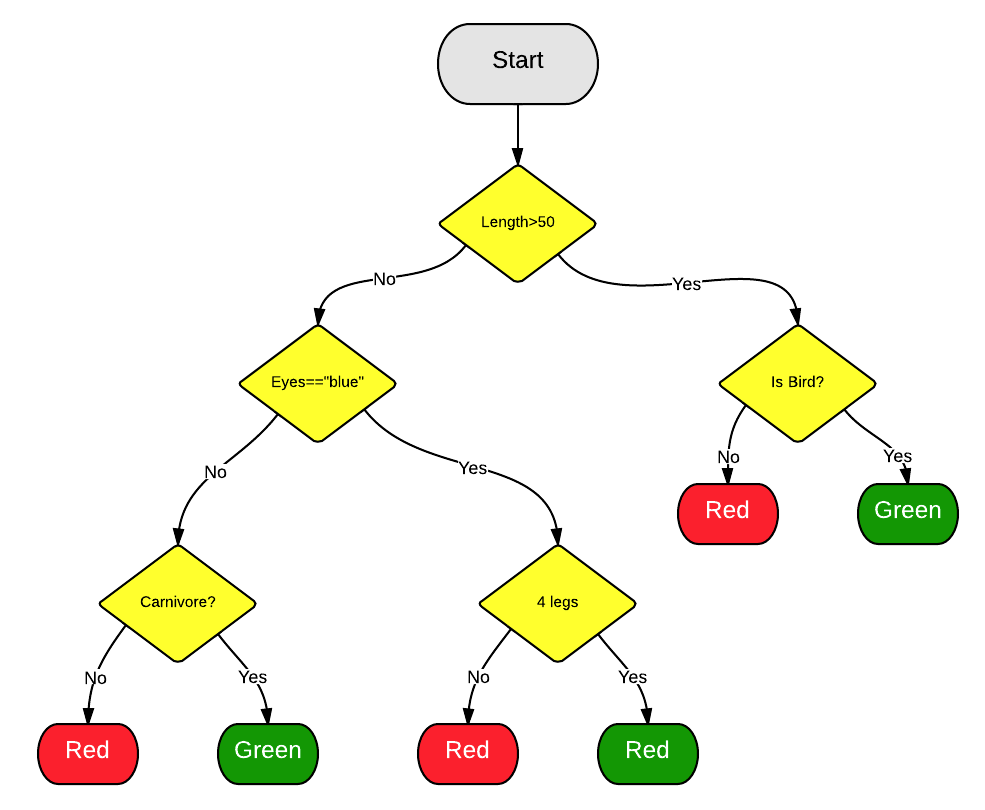###### 树的投票##### 为什么你让我用它？
###### 简单

[译者注：Leatherman就是那家生产多功能折叠刀的公司，类似瑞士军刀]

###### 一个映射的例子

import numpy as np
x = np.random.uniform(1, 100, 1000)
y = np.log(x) + np.random.normal(0, .3, 1000)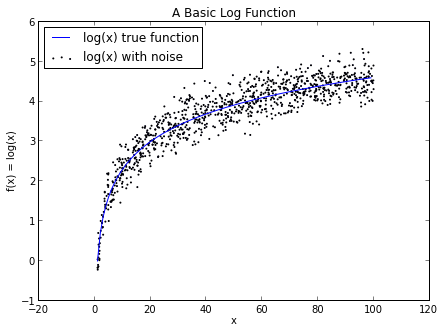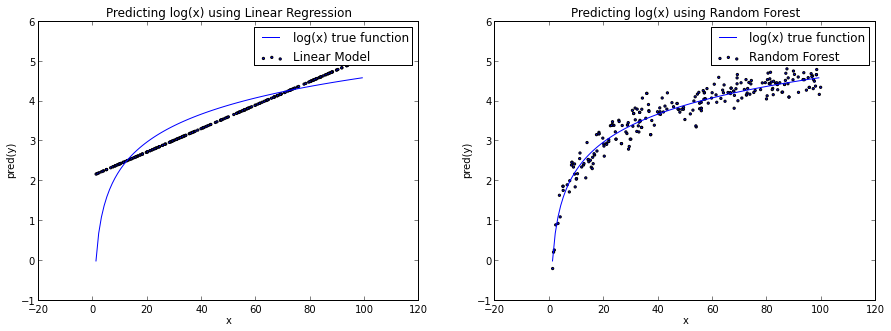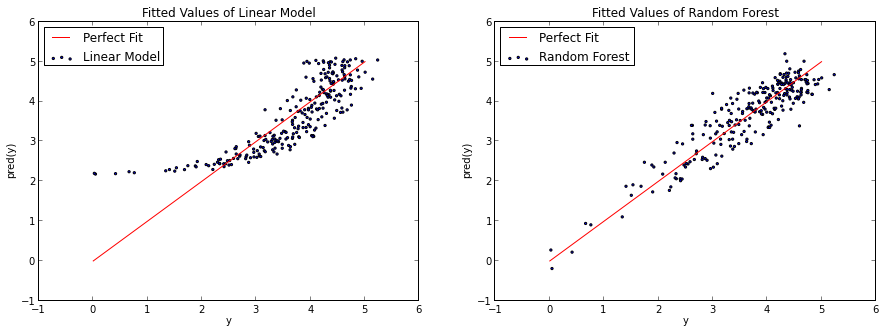##### 使用
###### 变量选择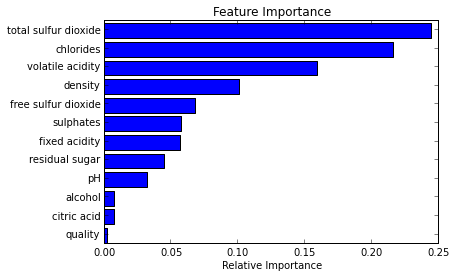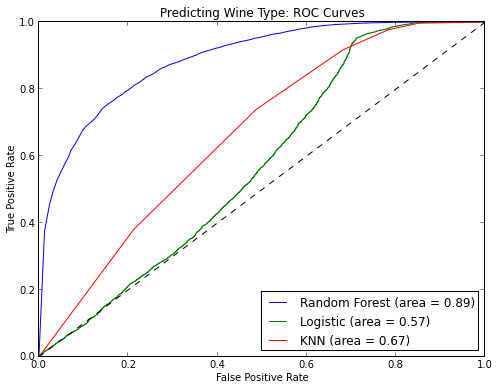##### 一个简短的python例子

Scikit-Learn是开始使用随机森林的一个很好的方式。scikit-learn API在所以算法中极其的一致，所有你测试和在不同的模型间切换非常容易。很多时候，我从一些简单的东西开始，然后转移到了随机森林。

from sklearn.datasets import load_iris
from sklearn.ensemble import RandomForestClassifier
import pandas as pd
import numpy as np
iris = load_iris()
df = pd.DataFrame(iris.data, columns=iris.feature_names)
df['is_train'] = np.random.uniform(0, 1, len(df)) <= .75
df['species'] = pd.Factor(iris.target, iris.target_names)
df.head()
train, test = df[df['is_train']==True], df[df['is_train']==False]
features = df.columns[:4]
clf = RandomForestClassifier(n_jobs=2)
y, _ = pd.factorize(train['species'])
clf.fit(train[features], y)
preds = iris.target_names[clf.predict(test[features])]
pd.crosstab(test['species'], preds, rownames=['actual'], colnames=['preds'])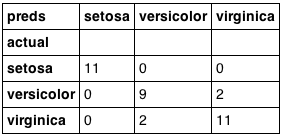##### 结语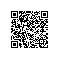使用钉钉扫一扫加入圈子
+ 订阅